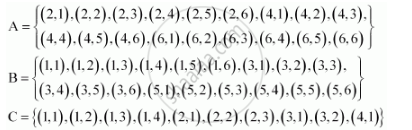# Two Dice Are Thrown. the Events A, B and C Are as Follows: a and C Are Mutually Exclusive - Mathematics

Two dice are thrown. The events A, B and C are as follows:

A: getting an even number on the first die.

B: getting an odd number on the first die.

C: getting the sum of the numbers on the dice ≤ 5

A and C are mutually exclusive

#### SolutionIt is observed that A ∩ C = {(2, 1), (2, 2), (2, 3), (4, 1)} ≠Φ

∴A and C are not mutually exclusive.

Thus, the given statement is false.

Concept: Event - Mutually Exclusive Events
Is there an error in this question or solution?
Chapter 16: Probability - Exercise 16.2 [Page 393]

#### APPEARS IN

NCERT Class 11 Mathematics
Chapter 16 Probability
Exercise 16.2 | Q 7.4 | Page 393

Share